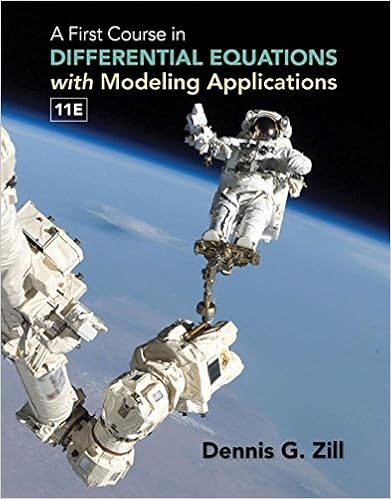# Solution is ˆ t ˆ t ˆ t a basis is 1 1 1 46 using

• Homework Help
• ChiefRockRaven1516
• 158
• 100% (1) 1 out of 1 people found this document helpful

This preview shows page 57 - 60 out of 158 pages.

##### We have textbook solutions for you!
The document you are viewing contains questions related to this textbook.The document you are viewing contains questions related to this textbook.
Chapter A / Exercise 14
A First Course in Differential Equations with Modeling Applications
ZillExpert Verified
Solution is: - ˆ t ˆ t ˆ t 0 , a basis is 1 1 1 0 . 46. Using Problem 45 find the general solution to the following linear system. 1 1 0 1 2 1 1 2 1 0 1 1 0 - 1 1 1 x y z w = 2 - 1 - 3 1 . Saylor URL: The Saylor Foundation
##### We have textbook solutions for you!
The document you are viewing contains questions related to this textbook.The document you are viewing contains questions related to this textbook.
Chapter A / Exercise 14
A First Course in Differential Equations with Modeling Applications
ZillExpert Verified
58 Exercises Solution is: - ˆ t ˆ t ˆ t 0 + - 9 5 0 6 , t R . 47. Find ker ( A ) for A = 1 2 3 2 1 0 2 1 1 2 1 4 4 3 3 0 2 1 1 2 . Recall ker ( A ) is just the set of solutions to A x = 0 . It is the solution space to the system A x = 0 . Solution is: w - v - 2 u - 1 2 u - 1 2 v - w u v w , u, v, w F . A basis is - 2 - 1 / 2 1 0 0 , - 1 - 1 / 2 0 1 0 , 1 - 1 0 0 1 48. Using Problem 47, find the general solution to the following linear system. 1 2 3 2 1 0 2 1 1 2 1 4 4 3 3 0 2 1 1 2 x 1 x 2 x 3 x 4 x 5 = 11 7 18 7 The augmented matrix is 1 2 3 2 1 11 0 2 1 1 2 7 1 4 4 3 3 18 0 2 1 1 2 7 , row echelon form: 1 0 2 1 - 1 4 0 1 1 2 1 2 1 7 2 0 0 0 0 0 0 0 0 0 0 0 0 . It follows that a particular solution is 4 7 / 2 0 0 0 . Then the general solution is s - 2 - 1 / 2 1 0 0 + t - 1 - 1 / 2 0 1 0 + w 1 - 1 0 0 1 + 4 7 / 2 0 0 0 49. Find the general solution to the following linear system. 1 2 3 2 1 0 2 1 1 2 1 4 4 3 3 0 2 1 1 2 x 1 x 2 x 3 x 4 x 5 = 6 7 13 7 Saylor URL: The Saylor Foundation
Exercises 59 50. Suppose A x = b has a solution. Explain why the solution is unique precisely when A x = 0 has only the trivial (zero) solution. If not, then there would be a infintely many solutions to A x = 0 and each of these added to a solution to A x = b would be a solution to A x = b . 51. Show that if A is an m × n matrix, then ker ( A ) is a subspace. If x , y ker ( A ) then A ( a x + b y ) = aA x + bA y = a 0 + b 0 = 0 and so ker ( A ) is closed under linear combinations. Hence it is a subspace. 52. Verify the linear transformation determined by the matrix of 9.2 maps R 3 onto R 2 but the linear transformation determined by this matrix is not one to one.
•••